### Fluorescence intensity decay models

#### Excited state decay of organic fluorophores

After electronic excitation the molecule typically decays to the lowest vibrational level of the first excited singlet state, S1. In far most cases, this happens non-radiatively in the order of pico-seconds by a combination of internal conversion (IC) and vibrational relaxation. From S1 a number of decay processes can occur to the electronic ground state, S0. If the oscillator strength of the S0-S1 transition is high the molecule may decay to the ground state via emission of radiation (fluorescence) and the molecule is thus termed a fluorophore.

The fluorescence, however, "competes" with non-radiative decay processes such as IC, intersystem crossing (ISC), or a bimolecular quenching process such as FRET and collisional quenching. Each process is associated with a rate constant, ki. The process with the largest value of ki dominates the decay. The total rate of decay from the excited state, then, is typically given by that of a unimolecular process: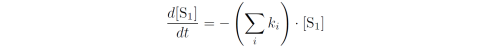where the sum is over all decay processes from the excited state. The solution to this 1. order differential equation iswhere [S1] is the concentration of fluorophores in S1 at time t and [S1]0 is the concentration of fluorophores in S1 at time t = 0. The lifetime of the fluorophore, τ, is defined as the time it takes a population of excited fluorophores to reach 1/e of [S1]0. Inserting this in the equation above and isolating t we get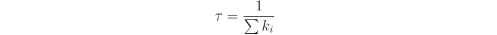where again the sum is over all decay processes from S1. The lifetime also corresponds to the average time that the molecule spends in the excited state.

Assuming that the fluorescence intensity is proportional to the excited state population, [S1], the intensity decay is exponential and given by:where I0 is the intensity at t = 0. If the sample possess more than one lifetime the intensity as a function of time is the sum of intensities from each decay: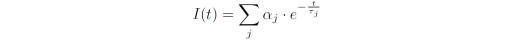which is called a multi-exponential decay. αj is the pre-exponential factor and can be used to represent the fraction of fluorophores with lifetime τj. The amplitude-weighted lifetime of the fluorophore is then given by: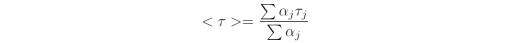Fluorescence decay models in DecayFit
In the DecayFit freeware the implemented intensity decay models are listed in the Fit models listbox:The intensity decay expression of the fit model selected in the listbox is shown below the listbox: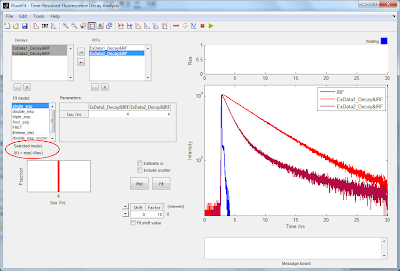A description of each of the fit models is provided below.

1. single_exp
A single-exponential decay corresponding to a single lifetime. Only the lifetime is provided as fit parameter.

2. double_exp
A double-exponential decay corresponding to two lifetimes. The sum of the pre-exponential factors is constrained to 1 which means that only one pre-factor, a1, is provided as fitting parameter while the other is set to be 1-a1.

3. triple_exp
A triple-exponential decay corresponding to three lifetimes. The sum of the pre-exponential factors are constrained to be a1+a2+a3 = 1.

4. four_exp
A four-exponential decay corresponding to four lifetimes. The sum of pre-exponential factors are constrained to be a1+a2+a3+a4 = 1.

5. FRET
A decay model for analyzing FRET data. The model implements a Gaussian distance distribution between the donor (D) and acceptor (A) with the distribution center (R-mean) and Full Width at Half Maximum (FWHM) as fitting parameters. The critical distance, R0, must be supplied as input and is not meant to be a fitting parameter (although this is possible by varying the min and max values).

Up to three lifetimes can be specified for the donor in absence of acceptor (tauD1, tauD2, tauD3), each with a pre-exponential factor (a1, a2, a3). These are not meant as fitting parameters but should be determined on a donor-only reference sample. If the donor has a single lifetime only set the min and max values of a2 and a3 to 0 (default).

The fraction of donors not coupled to an acceptor, f, can also be included as a parameter, either constrained or loosely fitted.

An additional component not involved in FRET with a lifetime 'tauB' and relative fraction 'b' can be included in the model. The fraction of the FRET-component in the decay, then, is 1-b.

Note: When using dynamic averaging, the D-A distance is modelled using a single distance - the mean of the Gaussian distribution (R-mean).

A Gaussian lifetime distribution model. A mixture of two independent Gaussians can be used, each with a lifetime center and a lifetime distribution width (FWHM) as fitting parameters.

7. double_exp_uncon
A double exponential decay in which there is no constraint on the sum of pre-exponential factors.

8. triple_exp_uncon
A triple exponential decay in which there is no constraint on the sum of pre-exponential factors.